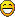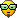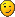## Turbulence and fluid mechanics

Post

### Turbulence and fluid mechanics

#1
Ars Technica just published this great article about turbulence. I am very glad to see this often underappreciated field of physics get such a well-written article!
Post

### Re: Turbulence and fluid mechanics

#2
Clear Air Turbulence?I love Foss ships!
Knowledge is a deadly friend, if no one sets the rules
The fate of all mankind, I see, is in the hands of fools
Post

### Re: Turbulence and fluid mechanics

#3
One of the most bizarre real-world things I ever saw was in my Fluid Dynamics class for Chemical Engineering, and the name of this freak of nature is the Reynolds number.

For those who haven't heard of this value, it's a quantity that describes when the flow of a stream changes from laminar to turbulent. So why is that weird?

Consider that most numbers that describe real-world things have some sort of dimensionality to them, which is expressed using some sort of units. Distances (one dimension) may be measured in cubits or feet or parsecs or ångstroms; areas (two dimensions) in square feet or hectares; volumes (three dimensions) in cubic centimeters; time in seconds; speed in meters per second (or furlongs/fortnight); acceleration in meters per second squared; force in newtons; frequencies in hertz; and and so on.

The Reynolds number has no dimension.

It's a real number, describing a real quantity: the fluid's speed with respect to an object in the stream (m/s), times a linear dimension (m), divided by the fluid's kinematic viscosity (m2/s), yielding a number that tells you when turbulent flow will happen. But the units cancel out! The number has no dimensionality of any kind. It's just a number. And yet it describes a real-world property that has real engineering utility.

There are some others, such as the Mach number. But I always found the Reynolds number particularly fascinating.

Yes, I probably should get out more.
Post

### Re: Turbulence and fluid mechanics

#6
In a way it makes sense though right? There's a text by Brian Cantwell that introduces the dimensional analysis chapter with,
Any physical relationship must be expressible in dimensionless form... This is because the variables are subject to measurement by an observer in terms of units that are selected at the arbitrary discretion of the observer. It is clear that a physical event ... cannot depend on the particular ruler used to measure space, the clock used for time, the scale used to measure mass... depending on the dimensions that appear in the problem.
If you and I run a race on a straight track that is 30 antehiros long, I run at an average pace of vScytale = 3 kilosquattes per hemtistool, and you run at an average pace of vFlatfingers = 49 terribads per naysec, who's going to win?

We don't know because we have no idea of the relative size of the measures we're using here, but we do know the winner depends on only these two quantities. The underlying principle of dimensional analysis is that, in this case, these two quantities can be collapsed into one because they have the same dimension: length per time. That means we can form a dimensionless number, call it the Flatfingers number,

Fa = vScytale / vFlatfingers

And so we can see that the winner of the race depends not on *two* quantities, but on one: the Flatfingers number. I don't know how big a kilosquatte is, and I don't know how long a naysec takes, but I know there are three solutions: if Fa > 1, I win: if Fa < 1, you win. If Fa = 1, it's a tie. In fact, Fa=1 is degenerate ("we both win").

Of course, to determine the Flatfingers number I need to know the conversion factors between the various units cited, so this all seems rather trivial. We've just shifted the problem into different language. But the language makes very clear what it is we should care about: it doesn't matter how fast I run, it only matters if I run faster than you.
If Fa is huge, it means I'm a speed demon compared to you, and if Fa is tiny, it means I'm a dismal failure compared to you.

We could say the Flatfingers number describes the "relative effects" of Scytale vs Flatfingers in the race. This is exactly the same language people use to talk about things like the Reynolds number: it describes the relative effects of inertia vs viscosity. And we know it works because it's dimensionless. If it weren't dimensionless, we couldn't know in general if inertia and viscosity were being compared on the same terms.

This is what makes these numbers so useful: Bond number is relative effects of surface tension vs gravity. Froude number, inertia/gravity, and so on.

Thank goodness for dimensionless numbers! If it weren't for Reynolds number, we couldn't describe this lab photo in exactly the same terms as this satellite view . Same relative effects, even though our measuring sticks are different.
Post

### Re: Turbulence and fluid mechanics

#7
From what I've read, relativity (in the general sense, not the Einsteinian sense) does seem to be the key to a particular class of dimensionlessness values.

But apparently there are also some dimensionless numbers that really do describe fundamental quantities of the physical universe.

Reality is weird.Post

### Re: Turbulence and fluid mechanics

#8
Flatfingers wrote:
Thu Oct 11, 2018 11:49 pm

The Reynolds number has no dimension.
Actually, this is not surprising, but the whole point.

Engineers (mostly chemical engineers, but some others as well) know that they cannot calculate from first principles.
But we need to calculate (I routinely in my job need to scale things up or down factor 10000, so 1L to 10m3).

How to compare different sizes when the effects are not being described by solvable equations?
Similar: how to compare different designs, specific designs, in this situation?

The answer is: try to abstract information in a size and specifics independent way (--> dimensionless), if possible in a way that have some intuitive meaning; then measure what you need and plot it versus this abstraction, or measure it vs. another abstraction, and make a model to fit the curve.
Then the idea is you can use the same curve/model for things that have different sizes or specifics in analogy and this will help you massively: somebody in literature measure stuff once with their system (or few systems) and I can use his work to describe many systems. In older times, there where books full of such curves (abacus) and equations.

Based on this, you can make many number; very used are for instance:
• Divide a force (e.g. inertial force due to current, speed of liquid) by another (e.g. viscous force) and you get a number showing the strength of the current vs the tendency to move around in turbulence, i.e. a description of the flow (laminar, no turbulence, vs turbulent). This is your Reynolds number Re. A low value means the inertial force is not large enough to win against the viscosity, hence the flow is laminar (no eddies), while a large value means the opposite (many eddies, meaning also that you need more power to move the liquid as eddies will dissipate power to heat).
• Heat transfer through a wall due to turbulence vs. heat transfer due to conduction = Nusselt number Nu.
• Heat transfer within a fluid due to turbulence vs. heat transfer due to diffusion = Prandtl number Pr
• Heat conduction * time - tendency to get rid of heat over (W/m.K * s = J / m.K) divided by the tendency to keep heat locally (cp * rho = J/kg.K * kg/m3 = J/K.m3) and as the conduction is in a direction while the heat storage is in a volume, let's divide by the square of the characteristic length, e.g. diameter of your pot (m2) . Now we get adimensional ( J / m.K * K.m3 / J * 1/m2) Fourier Number Fo.
And then, you ask? Well, it has been shown that in case of turbulent flow in tubes with smooth surface (most steel tubes, including in a heat exchanger):
Nu = 0.023 Re ^0.8 * Pr ^0.4
This is the sort of ugly correlation that we use. And guess what: Reynolds is easy to calculate / measure; so is Prandtl as it is inherent to a material and available for many substances in books. So now I can calculate Nusselt! And this allow me to calculate the not-easily-measurable heat transfer coefficient!
This is one of a zillion examples; and this equation "works" sort of as a good empirical approximation for different types of tubes, different materials flowing in the tubes and different sizes!

But you can make your own, if it is useful for you - let me put one quick stupid spontaneous example:
In conclusion: it is not surprising to land on an dimensionless number, it was by design chosen.
Second conclusion: don't let a chemical engineer begin ranting about dimensionless numbersPost

### Re: Turbulence and fluid mechanics

#9
Sure, the idea of inventing numbers to represent a ratio of relative quantities, and that a few of those relative quantities might be dimensionless, isn't completely whack. Just a bit interesting.

On the other hand, this article on the "fine structure constant" of the universe having a dimensionless value of 1/137 (which semi-randomly appeared in my feed today) sure seems peculiar to me, and to a few other people who understand this aspect of the world better than I do.

But go ahead; tell Richard Feynman his reaction to the weirdness of this value was exaggerated....Post

### Re: Turbulence and fluid mechanics

#10
Yes indeed, those fundamental constants are very strange. Very different footing from the idea of dimensionless numbers otherwise. What makes dimensional analysis so powerful is ultimately a kind of physical intuition about proportions. These mysterious proportionality constants though... no relation to that kind of thing! They're just there.

I'm double-checking my doors are locked tonight

### Online Now

Users browsing this forum: No registered users and 1 guest